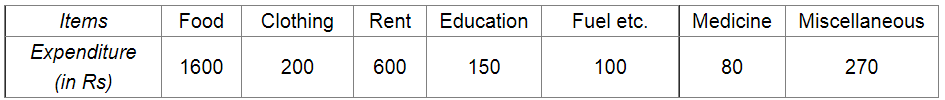# Following is the break up of the expenditure of a family on different items of consumption:

Question:

Following is the break up of the expenditure of a family on different items of consumption:Draw a pie-diagram to represent the above data.

Solution:

We know:

Central angle of a component $=\left(\right.$ component value $/$ sum of component values $\left.\times 360^{\circ}\right)$

Here, total expenditure = Rs 3000

Thus, central angle for each component can be calculated as follows:

 Item Expenditure (in Rs) Sector angle Food 1600 1600/3000 × 360 = 192 Clothing 200 200/3000 × 360 = 24 Rent 600 600/3000 × 360 = 72 Education 150 150/3000× 360 = 18 Fuel etc 100 100/3000 ×× 360 = 12 Medicine 80 80/3000 × 360 = 9.6 Miscellaneous 270 270/3000 × 360 = 32.4

Total : 3000 (in Rs)

Now, the pie chat representing the given data can be constructed by following the steps below:

Step 1 : Draw a circle of an appropriate radius.

Step 2 : Draw a vertical radius of the circle drawn in step 1.

Step 3 : Choose the largest central angle. Here, the largest central angle is 192o. Draw a sector with the central angle 192o in such a way that one of its radii coincides with the radius drawn in step 2 and another radius is in its counter clockwise direction.

Step 4 : Construct other sectors representing the other items in the clockwise sense in descending order of magnitudes of their central angles.

Step 5 : Shade the sectors with different colours and label them as shown in the figure below.​​